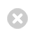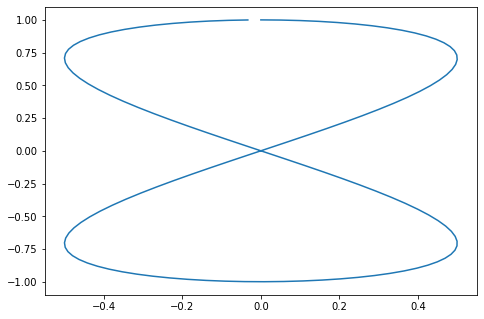Close

MPHY0021: Research Software Engineering With Python

Home# Introduction to Python¶

## Introduction¶

### Why teach Python?¶

• In this first session, we will introduce Python.
• This course is about programming for data analysis and visualisation in research.
• It's not mainly about Python.
• But we have to use some language.

### Why Python?¶

• Python is quick to program in
• Python is popular in research, and has lots of libraries for science
• Python interfaces well with faster languages
• Python is free, so you'll never have a problem getting hold of it, wherever you go.

### Why write programs for research?¶

• Not just labour saving
• Scripted research can be tested and reproduced

### Sensible Input - Reasonable Output¶

Programs are a rigorous way of describing data analysis for other researchers, as well as for computers.

Computational research suffers from people assuming each other's data manipulation is correct. By sharing codes, which are much more easy for a non-author to understand than spreadsheets, we can avoid the "SIRO" problem. The old saw "Garbage in Garbage out" is not the real problem for science:

• Sensible input
• Reasonable output

## Many kinds of Python¶

### The Jupyter Notebook¶

The easiest way to get started using Python, and one of the best for research data work, is the Jupyter Notebook.

In the notebook, you can easily mix code with discussion and commentary, and mix code with the results of that code; including graphs and other data visualisations.

In :
### Make plot
%matplotlib inline
import math

import numpy as np
import matplotlib.pyplot as plt

theta = np.arange(0, 4 * math.pi, 0.1)
eight = plt.figure()
axes = eight.add_axes([0, 0, 1, 1])
axes.plot(0.5 * np.sin(theta), np.cos(theta / 2))

Out:
[<matplotlib.lines.Line2D at 0x7fd2719185e0>]These notes are created using Jupyter notebooks and you may want to use it during the course. However, Jupyter notebooks won't be used for most of the activities and exercises done in class. To get hold of a copy of the notebook, follow the setup instructions shown on the course website, or use the installation in Desktop@UCL (available in the teaching cluster rooms or anywhere).

Jupyter notebooks consist of discussion cells, referred to as "markdown cells", and "code cells", which contain Python. This document has been created using Jupyter notebook, and this very cell is a Markdown Cell.

In :
print("This cell is a code cell")

This cell is a code cell


Code cell inputs are numbered, and show the output below.

Markdown cells contain text which uses a simple format to achive pretty layout, for example, to obtain:

bold, italic

• Bullet

Quote

We write:

**bold**, *italic*

* Bullet

> Quote



See the Markdown documentation at This Hyperlink

### Typing code in the notebook¶

When working with the notebook, you can either be in a cell, typing its contents, or outside cells, moving around the notebook.

• When in a cell, press escape to leave it. When moving around outside cells, press return to enter.
• Outside a cell:
• Use arrow keys to move around.
• Press b to add a new cell below the cursor.
• Press m to turn a cell from code mode to markdown mode.
• Press shift+enter to calculate the code in the block.
• Press h to see a list of useful keys in the notebook.
• Inside a cell:
• Press tab to suggest completions of variables. (Try it!)

### Python at the command line¶

Data science experts tend to use a "command line environment" to work. You'll be able to learn this at our "Software Carpentry" workshops, which cover other skills for computationally based research.

In :
%%bash
# Above line tells Python to execute this cell as *shell code*
# not Python, as if we were in a command line
# This is called a 'cell magic'

python -c "print(2 * 4)"

8


### Python scripts¶

Once you get good at programming, you'll want to be able to write your own full programs in Python, which work just like any other program on your computer. Here are some examples:

In :
%%bash
echo "print(2 * 4)" > eight.py
python eight.py

8


We can make the script directly executable (on Linux or Mac) by inserting a hashbang and setting the permissions to execute.

In :
%%writefile fourteen.py
#! /usr/bin/env python
print(2 * 7)

Writing fourteen.py

In :
%%bash
chmod u+x fourteen.py
./fourteen.py

14


### Python Libraries¶

We can write our own python libraries, called modules which we can import into the notebook and invoke:

In :
%%writefile draw_eight.py
# Above line tells the notebook to treat the rest of this
# cell as content for a file on disk.
import math

import numpy as np
import matplotlib.pyplot as plt

def make_figure():
theta = np.arange(0, 4 * math.pi, 0.1)
eight = plt.figure()
axes = eight.add_axes([0, 0, 1, 1])
axes.plot(0.5 * np.sin(theta), np.cos(theta / 2))
return eight

Writing draw_eight.py


In a real example, we could edit the file on disk using a program such as Atom or VS code.

In :
import draw_eight # Load the library file we just wrote to disk

In :
image = draw_eight.make_figure()There is a huge variety of available packages to do pretty much anything. For instance, try import antigravity.

The %% at the beginning of a cell is called magics. There's a large list of them available and you can create your own.